# Midterm 2 Preparation

The second midterm will be composed of fifteen questions covering chapters 5 to 7.

Twelve questions will be algebra questions, and three questions will be word problems.

• Questions 1–4 will be drawn from Chapter 5: Systems of Equations
• 5.1 Graphed Solutions
• 5.2 Substitution Solutions
• 5.3 Addition and Subtraction Solutions
• 5.4 Solving for Three Variables
• Questions 5–6 will be drawn from Chapter 6: Polynomials
• 6.1 Working with Exponents
• 6.2 Negative Exponents
• 6.3 Scientific Notation
• 6.4 Basic Operations Using Polynomials
• 6.5 Multiplication of Polynomials
• 6.6 Special Products
• 6.7 Dividing Polynomials
• Questions 9–12 will be drawn from Chapter 7: Factoring
• 7.1 Greatest Common Factor
• 7.2 Factoring by Grouping
• 7.3 Factoring Trinomials where a = 1
• 7.4 Factoring Trinomials where a ≠ 1
• 7.5 Factoring Special Products
• 7.6 Factoring Quadratics of Increasing Difficulty
• 7.7 Choosing the Correct Factoring Strategy
• 7.8 Solving Quadratic Equations by Factoring
• Questions 13–15 will be drawn from:
• 5.5 Monetary Word Problems
• 6.8 Mixture and Solution Word Problems
• 7.9 Age Word Problems

Students will be allowed to use MATQ 1099 Data Booklets & Glossaries for both Midterms and the Final Exam.

# Midterm Two Review

## Chapter 4: Systems of Equations (Exam Type Questions)

Find the solution set of the system of equations below graphically.

1.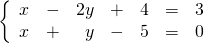For problems 2–4, find the solution set of each system by any convenient method.

1.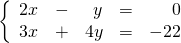2.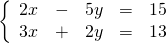3.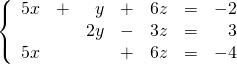## Chapter 5: Polynomials (Exam Type Questions)

Simplify.

1.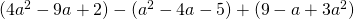2.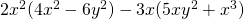3.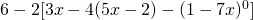4.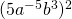5.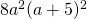6.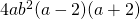7.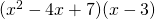8.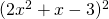9.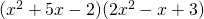10.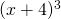11.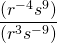Divide using long division.

1.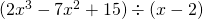## Chapter 6: Factoring (Exam Type Questions)

Questions 18–19 are for review only and will not show up on exams.

1. Find the prime factorization of 88.
2. Find the LCM of 84 and 96.

Questions similar to 20–27 will show up on exams.

Factor each expression completely.

1.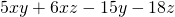2.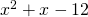3.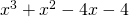4.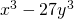5.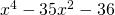## Chapter 4–6 Word Problems (Exam Type Questions)

1. A 70 kg mixture of two different types of nuts costs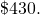If type A costs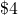per kg and type B costs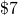per kg, how many kg of each type were used?
2. A wedding reception bartender is faced with a problem: how many litres of a 5% rum drink must be mixed with 2 litres of a 21% flavoured rum to make a rum punch of 11% strength?
3. The sum of the ages of a boy and a girl is 16 years. Four years ago, the girl was three times the age of the boy. Find the present age of each child.

<a class=”internal” href=”/intermediatealgebraberg/back-matter/midterm-2-prep-answer-key/”>Mid Term 2 Prep Answer Key